# 支持向量机 (SVM)

SVM也称为边缘分类器，因为它在类之间绘制边缘。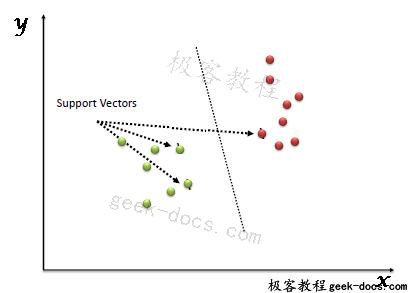## Simple SVM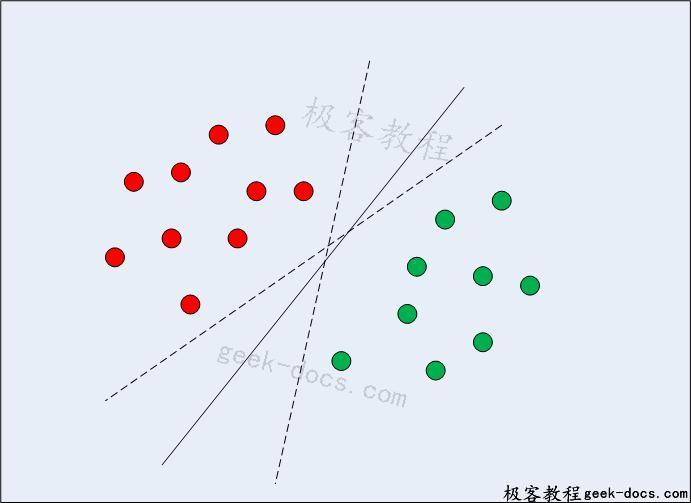SVM与其他分类算法的不同之处在于，它选择的决策边界使所有类与最近数据点的距离最大。支持向量机不仅仅是找到一个决策边界;而且找到最优决策边界。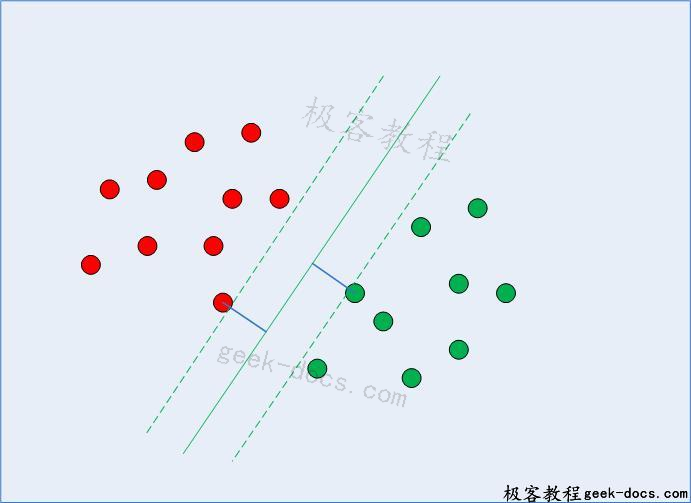## 用Scikit-Learn实现SVM

import pandas as pd
import numpy as np
import matplotlib.pyplot as plt
%matplotlib inline


https://archive.ics.uci.edu/ml/datasets/banknote+authentication

bankdata = pd.read_csv("D:/Datasets/bill_authentication.csv")


bankdata.shape


bankdata.head()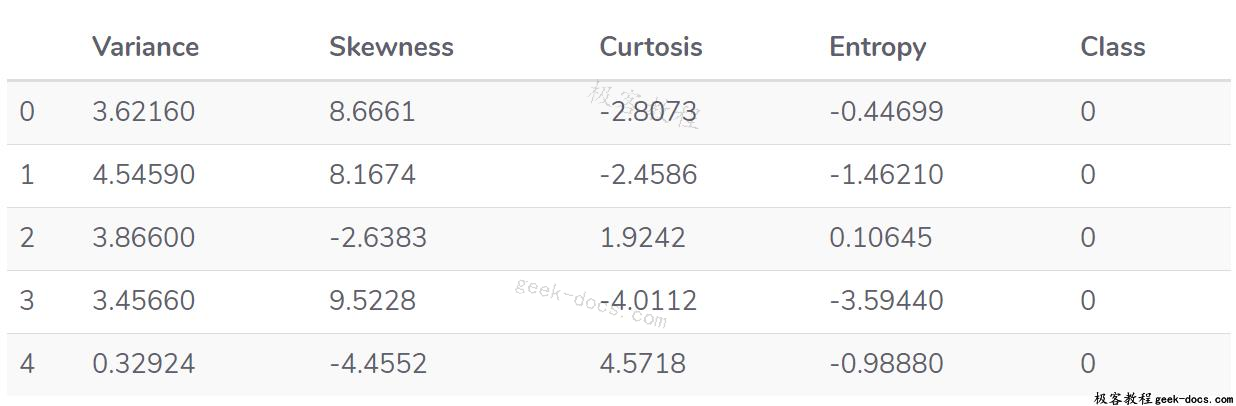X = bankdata.drop('Class', axis=1)
y = bankdata['Class']


from sklearn.model_selection import train_test_split
X_train, X_test, y_train, y_test = train_test_split(X, y, test_size = 0.20)


from sklearn.svm import SVC
svclassifier = SVC(kernel='linear')
svclassifier.fit(X_train, y_train)


y_pred = svclassifier.predict(X_test)


from sklearn.metrics import classification_report, confusion_matrix
print(confusion_matrix(y_test,y_pred))
print(classification_report(y_test,y_pred))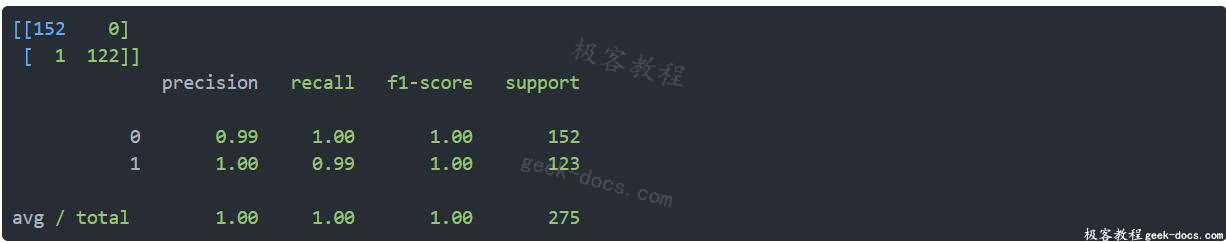• 回顶